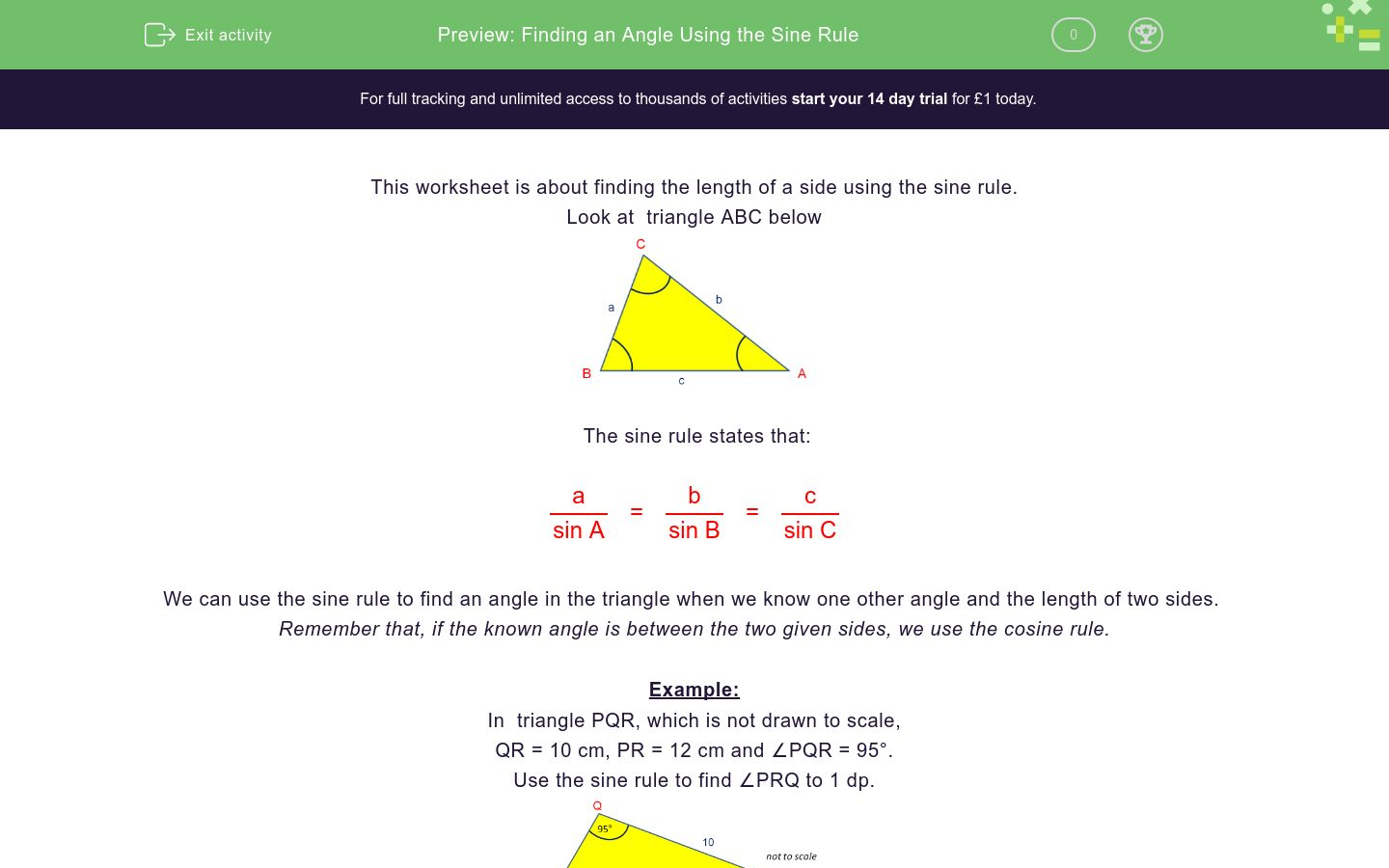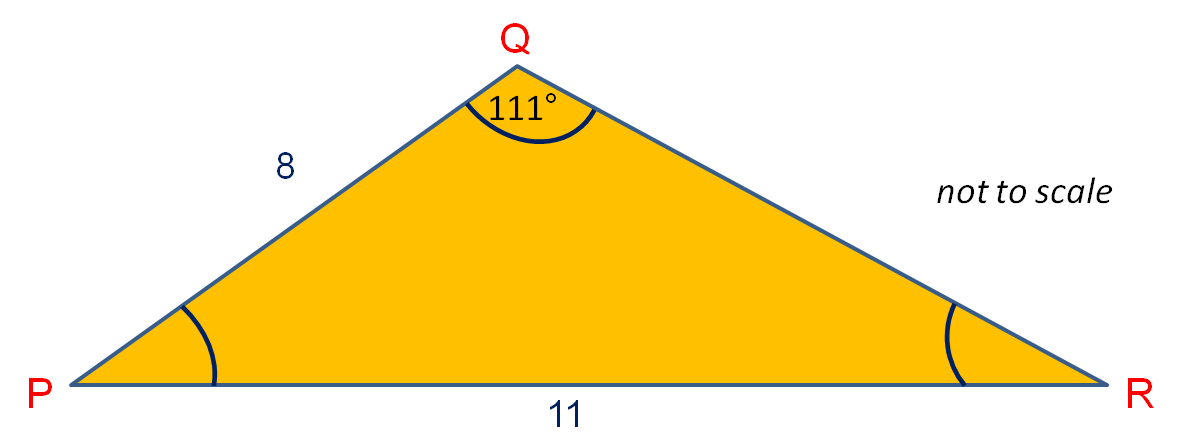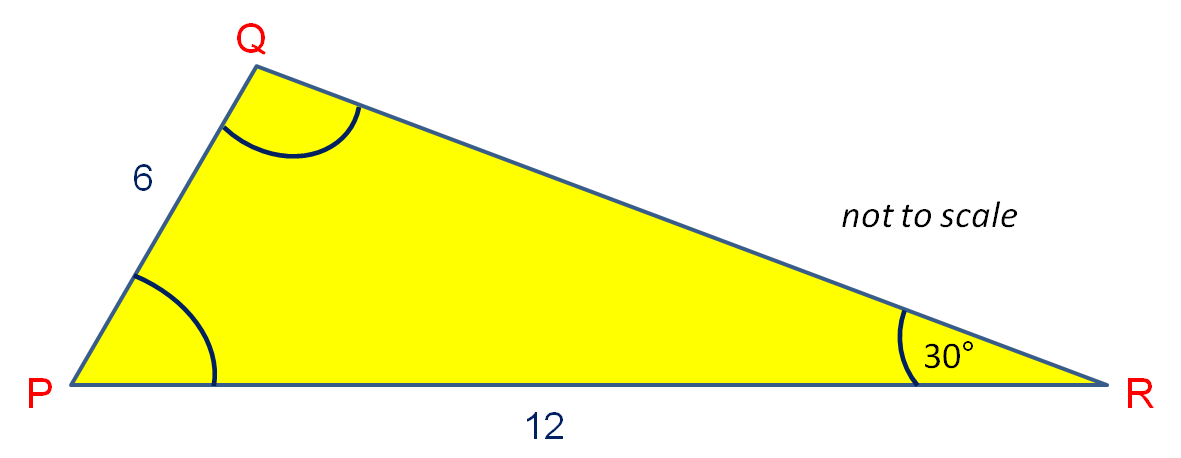# Finding an Angle Using the Sine Rule

In this worksheet, students find an angle given one angle and two sides in a triangle by using the sine rule.Key stage:  KS 4

Curriculum topic:  Geometry and Measures

Difficulty level:### QUESTION 1 of 10

This worksheet is about finding the length of a side using the sine rule.

Look at  triangle ABC belowThe sine rule states that:

 a = b = c sin A sin B sin C

We can use the sine rule to find an angle in the triangle when we know one other angle and the length of two sides.

Remember that, if the known angle is between the two given sides, we use the cosine rule.

Example:

In  triangle PQR, which is not drawn to scale,

QR = 10 cm, PR = 12 cm and ∠PQR = 95°.

Use the sine rule to find ∠PRQ to 1 dp.First we must find ∠QPR using the sine rule.

Using the sine rule,

 12 = 10 sin 95° sin ∠QPR

 sin ∠QPR = 10 x sin 95° 12

sin ∠QPR = 0.83016...

∠QPR = sin-1 (0.83016) = 56.1°

∠PQR = 180° - 56.1° - 95° = 28.9°

In  triangle PQR, which is not drawn to scale,

QR = 8 cm, PR = 10 cm and ∠PQR = 97°

Use the sine rule to find ∠QPR to 1 dp.

(Just write the number)In  triangle PQR, which is not drawn to scale,

PQ = 5 cm, PR = 10 cm and ∠PQR = 93°

Use the sine rule to find ∠QRP to 1 dp.

(Just write the number)In  triangle PQR, which is not drawn to scale,

PQ = 9 cm, PR = 15 cm and ∠QRP = 37°

Use the sine rule to find ∠PQR to 1 dp.

(Just write the number)In  triangle PQR, which is not drawn to scale,

PQ = 9 cm, QR = 10 cm and ∠QRP = 37°

Use the sine rule to find ∠PQR to 1 dp.

(Just write the number)In  triangle PQR, which is not drawn to scale,

PQ = 9 cm, QR = 10 cm and ∠QPR = 41°

Use the sine rule to find ∠QRP to 1 dp.

(Just write the number)In  triangle PQR, which is not drawn to scale,

PQ = 14 cm, QR = 10 cm and ∠QPR = 41°

Use the sine rule to find ∠PQR to 1 dp.

(Just write the number)In  triangle PQR, which is not drawn to scale,

PQ = 14 cm, PR = 10 cm and ∠PRQ = 123°

Use the sine rule to find ∠QPR to 1 dp.

(Just write the number)In  triangle PQR, which is not drawn to scale,

QR = 7.5 cm, PR = 10 cm and ∠PQR = 40°

Use the sine rule to find ∠QPR to 1 dp.

(Just write the number)In  triangle PQR, which is not drawn to scale,

PQ = 8 cm, PR = 11 cm and ∠PQR = 111°

Use the sine rule to find ∠QPR to 1 dp.

(Just write the number)In  triangle PQR, which is not drawn to scale,

PQ = 6 cm, PR = 12 cm and ∠QRP = 30°

Use the sine rule to find ∠PQR.

(Just write the number)• Question 1

In  triangle PQR, which is not drawn to scale,

QR = 8 cm, PR = 10 cm and ∠PQR = 97°

Use the sine rule to find ∠QPR to 1 dp.

(Just write the number)52.6
EDDIE SAYS
 10 = 8 sin 97° sin ∠QPR
• Question 2

In  triangle PQR, which is not drawn to scale,

PQ = 5 cm, PR = 10 cm and ∠PQR = 93°

Use the sine rule to find ∠QRP to 1 dp.

(Just write the number)30.0
EDDIE SAYS
 10 = 5 sin 93° sin ∠QRP
• Question 3

In  triangle PQR, which is not drawn to scale,

PQ = 9 cm, PR = 15 cm and ∠QRP = 37°

Use the sine rule to find ∠PQR to 1 dp.

(Just write the number)60.4
EDDIE SAYS
 9 = 13 sin 37° sin ∠PQR
• Question 4

In  triangle PQR, which is not drawn to scale,

PQ = 9 cm, QR = 10 cm and ∠QRP = 37°

Use the sine rule to find ∠PQR to 1 dp.

(Just write the number)101.0
EDDIE SAYS
 9 = 10 sin 63° sin ∠QPR

∠PQR = 180° - 37° - ∠QPR
• Question 5

In  triangle PQR, which is not drawn to scale,

PQ = 9 cm, QR = 10 cm and ∠QPR = 41°

Use the sine rule to find ∠QRP to 1 dp.

(Just write the number)36.2
EDDIE SAYS
 10 = 9 sin 41° sin ∠QRP
• Question 6

In  triangle PQR, which is not drawn to scale,

PQ = 14 cm, QR = 10 cm and ∠QPR = 41°

Use the sine rule to find ∠PQR to 1 dp.

(Just write the number)72.3
EDDIE SAYS
 10 = 14 sin 41° sin ∠QRP

∠PQR = 180° - 41° - ∠QRP
• Question 7

In  triangle PQR, which is not drawn to scale,

PQ = 14 cm, PR = 10 cm and ∠PRQ = 123°

Use the sine rule to find ∠QPR to 1 dp.

(Just write the number)20.2
EDDIE SAYS
 14 = 10 sin 123° sin ∠PQR

∠QPR = 180° - 123° - ∠PQR
• Question 8

In  triangle PQR, which is not drawn to scale,

QR = 7.5 cm, PR = 10 cm and ∠PQR = 40°

Use the sine rule to find ∠QPR to 1 dp.

(Just write the number)28.8
EDDIE SAYS
 10 = 7.5 sin 40° sin ∠QPR
• Question 9

In  triangle PQR, which is not drawn to scale,

PQ = 8 cm, PR = 11 cm and ∠PQR = 111°

Use the sine rule to find ∠QPR to 1 dp.

(Just write the number)26.2
EDDIE SAYS
 11 = 8 sin 111° sin ∠QRP

∠QPR = 180° - 111° - ∠QRP
• Question 10

In  triangle PQR, which is not drawn to scale,

PQ = 6 cm, PR = 12 cm and ∠QRP = 30°

Use the sine rule to find ∠PQR.

(Just write the number)90
90.0
EDDIE SAYS
 6 = 12 sin 30° sin ∠PQR

This is a right-angled triangle
---- OR ----

Sign up for a £1 trial so you can track and measure your child's progress on this activity.

### What is EdPlace?

We're your National Curriculum aligned online education content provider helping each child succeed in English, maths and science from year 1 to GCSE. With an EdPlace account you’ll be able to track and measure progress, helping each child achieve their best. We build confidence and attainment by personalising each child’s learning at a level that suits them.

Start your £1 trial